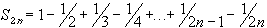#Interactive Real Analysis

Next | Previous | Glossary | Map

## 4.3. Special Series

### Definition: Alternating Harmonic Series

The seriesis called the Alternating Harmonic series. It converges but not absolutely, i.e. it converges conditionally.

### You browser is not 'Java-enabled' ...

There are many proofs of this fact. For example. the series of absolute values is a p-series with p = 1, and diverges by the p-series test. The original series converges, because it is an alternating series, and the alternating series test applies easily. However, here is a more elementary proof of the convergence of the alternating harmonic series.

We already know that the series of absolute values does not converge by a previous example. Hence, the series does not converge absolutely. As for regular convergence, consider the following two partial sums:andWe have that
S 2n+2 - S 2n = 1 / (2n+1) - 1 / (2n+2) > 0
and
S 2n+3 - S 2n+1 = - 1 / (2n+2) + 1/ (2n+3) < 0
which means for the two subsequences
{ S 2n } is monotone increasing and { S 2n+1 } is monotone decreasing
For each sequence we can combine pairs to see that
S 2n1 and S 2n+10
for all n. Hence, both subsequences are monotone and bounded and must therefore be convergent. Define their limits as
lim S 2n = L and lim S 2n+1 = M
Then
| M - L | = | lim (S 2n+1 - S 2n) | = 1 / (2n+1)
which converges to zero. Therefore, M = L, i.e. both subsequences converge to the same limit. But this common limit is the same as the limit of the full sequence, because: given any> 0 we have
• there exists an integer N such that | L - S 2n | <if n > N
• there exists an integer M such that | L - S 2n+1 | <if n > M
Now set K = max(N, M). Then, for the above> 0 we have
| L - S n | <for n > K because n is either even or odd. Hence, the alternating harmonic series converges conditionally.Next | Previous | Glossary | Map